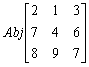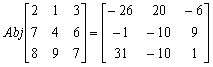3x3伴隨矩陣計算2 = 1 = 3 = =

7 = 4 = 6 = =

8 = 9 = 7 = =

= = = (顯示det=40) =

= = = 40 =

= = = 0 =

= = = 0 = (顯示x=-26) = (顯示y=-1) = (顯示z=31) = =

= = = 0 =

= = = 40 =

= = = 0 =

(顯示x=20) = (顯示y=-10) = (顯示z=-10) = =

= = = 0 =

= = = 0 =

= = = 40 = (顯示x=-6) = (顯示y=9) = (顯示z=1) =3x3逆矩陣的計算 (應用內置聯立三元一次方程 ) (3 × 3 Inverse matrix)

3x3逆矩陣乘法 (應用內置聯立三元一次方程 ) (3 × 3 Inverse matrix multiplication)